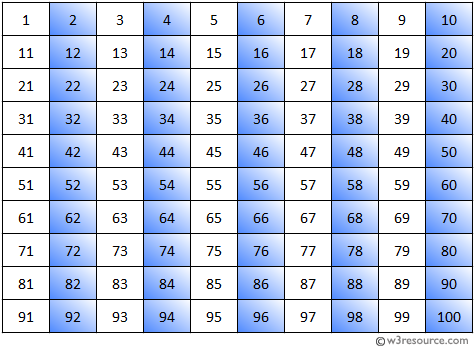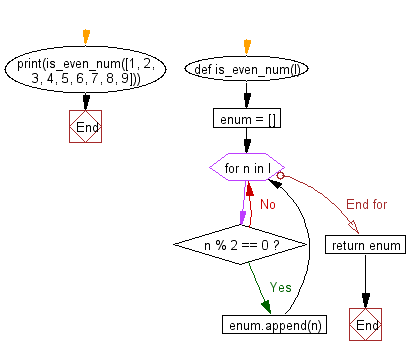﻿ Python Exercise: Print the even numbers from a given list - w3resource# Python Exercise: Print the even numbers from a given list

## Python Functions: Exercise-10 with Solution

Write a Python program to print the even numbers from a given list.Sample Solution:-

Python Code:

``````def is_even_num(l):
enum = []
for n in l:
if n % 2 == 0:
enum.append(n)
return enum
print(is_even_num([1, 2, 3, 4, 5, 6, 7, 8, 9]))
```
```

Sample Output:

```[2, 4, 6, 8]
```

Flowchart:## Visualize Python code execution:

The following tool visualize what the computer is doing step-by-step as it executes the said program:

Python Code Editor:

Have another way to solve this solution? Contribute your code (and comments) through Disqus.

What is the difficulty level of this exercise?

Test your Programming skills with w3resource's quiz.

﻿

## Python: Tips of the Day

Decapitalizes the first letter of a string:

Example:

```def tips_decapitalize(s, upper_rest=False):
return s[:1].lower() + (s[1:].upper() if upper_rest else s[1:])
print(tips_decapitalize('PythonTips'))
print(tips_decapitalize('PythonTips', True))
```

Output:

```pythonTips
pYTHONTIPS
```